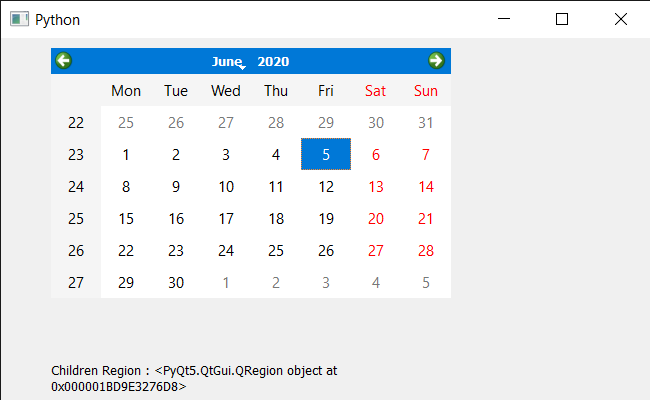# PyQt5 QCalendarWidget – Accessing Children Region

• Last Updated : 05 Jan, 2022

In this article we will see how we get the children region of the QCalendarWidget. Calendar is not alone a single widget it is a mixture of lots of smaller widget which we refer as calendar’s child. There are many child such as table view, item delegate etc, we use `children` method to get all the children of it. Children region is the region(area occupied) of all the children except hidden children i.e combined region of all the widgets.

In order to do this we will use `childrenRegion` method with the QCalendarWidget object.

Syntax : calendar.childrenRegion()

Argument : It takes no argument

Return : It return QRegion object

Below is the implementation

## Python3

 `# importing libraries``from` `PyQt5.QtWidgets ``import` `*` `from` `PyQt5 ``import` `QtCore, QtGui``from` `PyQt5.QtGui ``import` `*` `from` `PyQt5.QtCore ``import` `*` `import` `sys`` ` ` ` `class` `Window(QMainWindow):`` ` `    ``def` `__init__(``self``):``        ``super``().__init__()`` ` `        ``# setting title``        ``self``.setWindowTitle(``"Python "``)`` ` `        ``# setting geometry``        ``self``.setGeometry(``100``, ``100``, ``650``, ``400``)`` ` `        ``# calling method``        ``self``.UiComponents()`` ` `        ``# showing all the widgets``        ``self``.show()`` ` `    ``# method for components``    ``def` `UiComponents(``self``):`` ` `        ``# creating a QCalendarWidget object``        ``self``.calendar ``=` `QCalendarWidget(``self``)`` ` `        ``# setting geometry to the calendar``        ``self``.calendar.setGeometry(``50``, ``10``, ``400``, ``250``)`` ` `        ``# creating a label``        ``label ``=` `QLabel(``self``)`` ` `        ``# setting geometry``        ``label.setGeometry(``50``, ``280``, ``420``, ``120``)`` ` `        ``# making it multi line``        ``label.setWordWrap(``True``)`` ` `        ``# getting children region ``        ``value ``=` `self``.calendar.childrenRegion()`` ` `        ``# setting text to the label``        ``label.setText(``"Children Region : "` `+` `str``(value))`` ` ` ` ` ` `# create pyqt5 app``App ``=` `QApplication(sys.argv)`` ` `# create the instance of our Window``window ``=` `Window()`` ` ` ` `# start the app``sys.exit(App.``exec``())`

Output :My Personal Notes arrow_drop_up